# Lectures 3 Monte Carlo method in finance Lab

• Slides: 11
Download presentationLectures 3 – Monte Carlo method in finance: Lab The goals: n create a VBA package/framework to price exotic options via Monte Carlo method and its modifications (i. e. variance reduction techniques). n create an excel file to be used as a front end for pricing options. 3/12/2021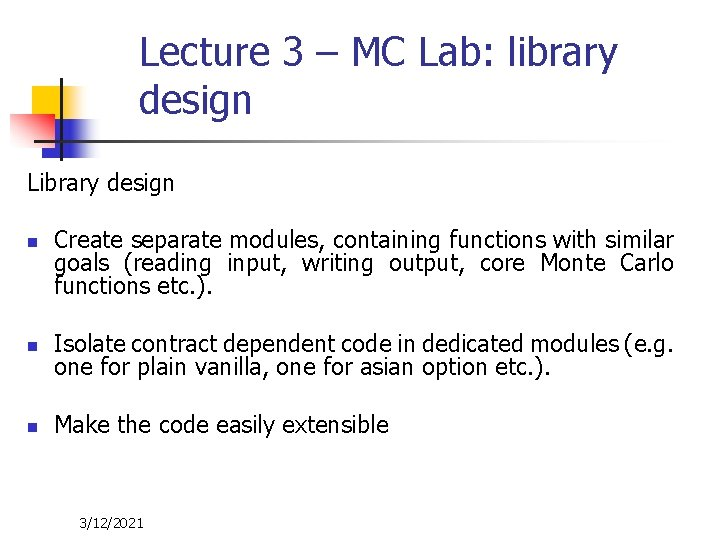Lecture 3 – MC Lab: library design Library design n Create separate modules, containing functions with similar goals (reading input, writing output, core Monte Carlo functions etc. ). n Isolate contract dependent code in dedicated modules (e. g. one for plain vanilla, one for asian option etc. ). n Make the code easily extensible 3/12/2021Lecture 3 – MC Lab: Excel as a front end (I). n define sheets to parameters (input): n n n manage Monte Carlo scenario numbers (N) flag for: activate antithetic sampling or control variates define sheets to store market data (input) n n Yield curves Equity data (current stock price, dividends, volatility) 3/12/2021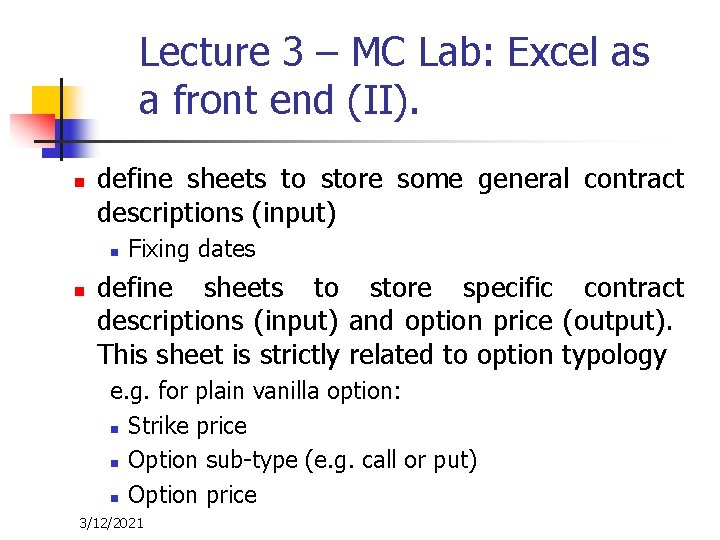Lecture 3 – MC Lab: Excel as a front end (II). n define sheets to store some general contract descriptions (input) n n Fixing dates define sheets to store specific contract descriptions (input) and option price (output). This sheet is strictly related to option typology e. g. for plain vanilla option: n Strike price n Option sub-type (e. g. call or put) n Option price 3/12/2021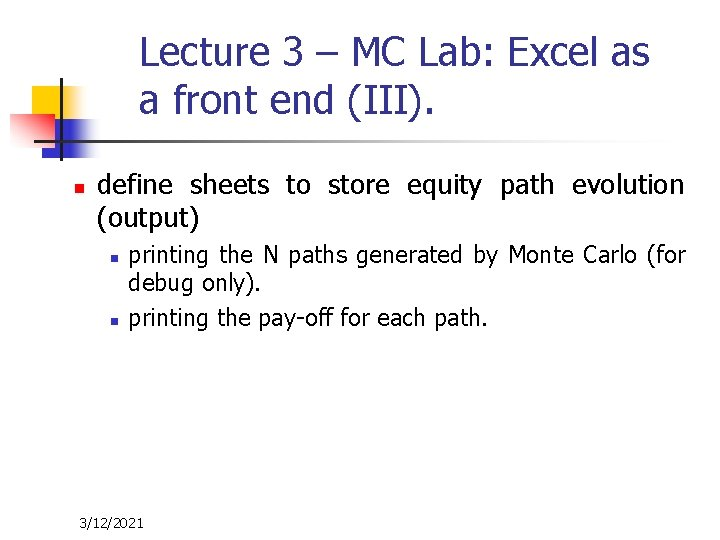Lecture 3 – MC Lab: Excel as a front end (III). n define sheets to store equity path evolution (output) n n printing the N paths generated by Monte Carlo (for debug only). printing the pay-off for each path. 3/12/2021Lecture 3 – MC Lab: VBA library (I). n Structures to manage: n n Fixing: future fixing dates Yield curve market data (rates for different maturities), Equity market data: price, dividend yield and volatility. Function to read from excel data to initialize the structures defined above. 3/12/2021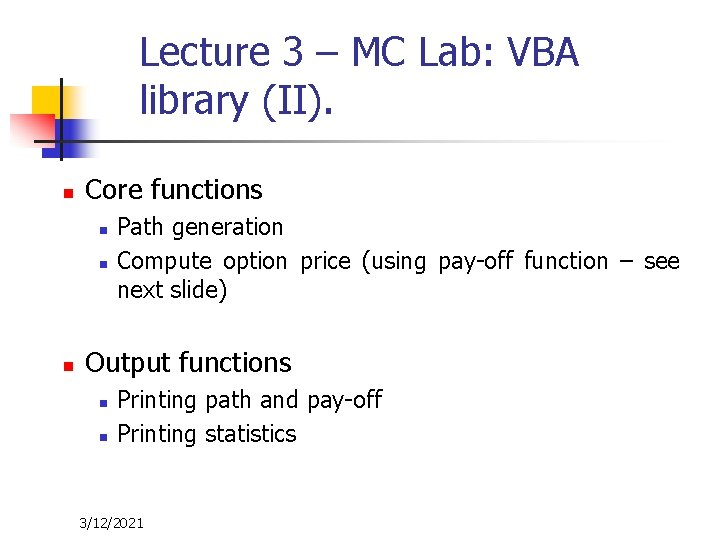Lecture 3 – MC Lab: VBA library (II). n Core functions n n n Path generation Compute option price (using pay-off function – see next slide) Output functions n n Printing path and pay-off Printing statistics 3/12/2021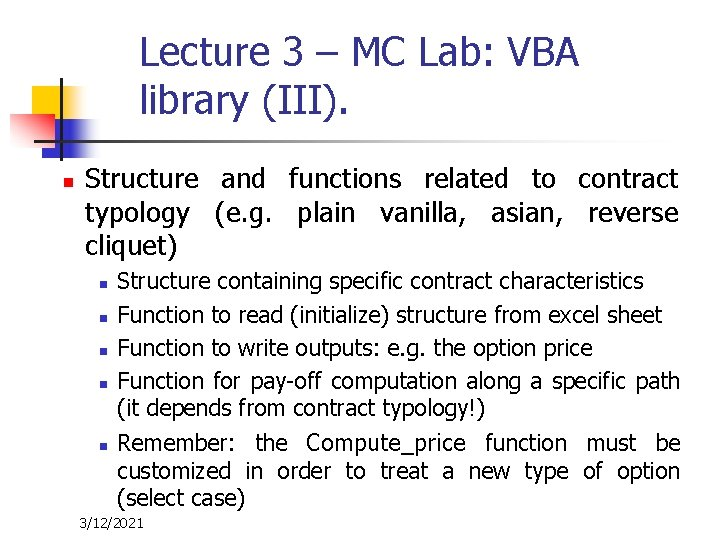Lecture 3 – MC Lab: VBA library (III). n Structure and functions related to contract typology (e. g. plain vanilla, asian, reverse cliquet) n n n Structure containing specific contract characteristics Function to read (initialize) structure from excel sheet Function to write outputs: e. g. the option price Function for pay-off computation along a specific path (it depends from contract typology!) Remember: the Compute_price function must be customized in order to treat a new type of option (select case) 3/12/2021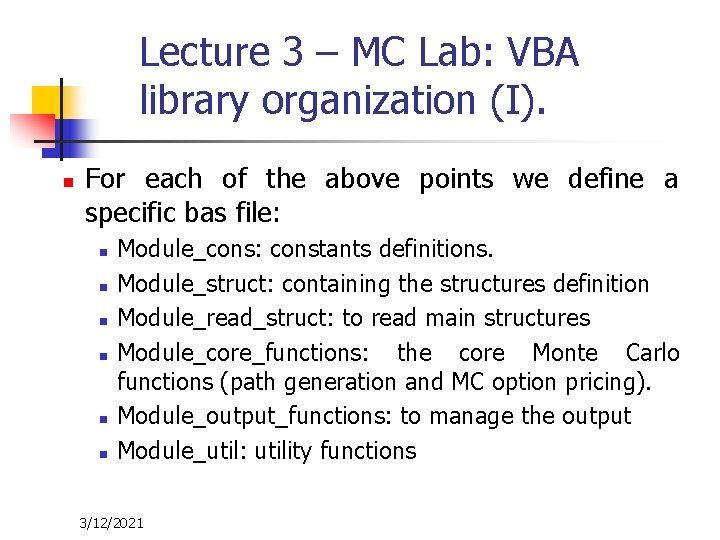Lecture 3 – MC Lab: VBA library organization (I). n For each of the above points we define a specific bas file: n n n Module_cons: constants definitions. Module_struct: containing the structures definition Module_read_struct: to read main structures Module_core_functions: the core Monte Carlo functions (path generation and MC option pricing). Module_output_functions: to manage the output Module_util: utility functions 3/12/2021Lecture 3 – MC Lab: VBA library organization (II). n n n Module_pv: containing structure and functions to manage plain vanilla options Module_asian: containing structure and functions to manage asian options Module_reverse_cliquet: containing structure and functions to manage reverse cliquet options 3/12/2021Lecture 3 – MC Lab: Exercises n n Ex. 1: verify that Monte Carlo error scales with N (the scenario’s number) as 1/sqrt(N). Suggestion: compute option prices with different values of N and plot the data in a log-log graph. Ex. 2: within VBA library, implement the pay-off calculation for a reverse cliquet option and compute, via Monte Carlo simulation, its value. n 3/12/2021 Suggestion: take the following parameters values n H = 4% n L = 0% n M=12, i. e. twelve fixing dates (with delta_t = 1/12 year)